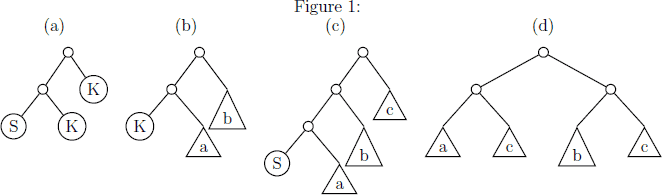## Day 1, Problem 3: S and K

You may be wondering what is the simplest computer we can create that can still perform useful computation. Over the years, theoretical computer scientists have devised various simple models of computation such as Turing machines and lambda calculus. In this problem, we will see how we can perform arbitrary computation in an even simpler system devised in 1924 by Moses Schönfinkel, using just the letters S and K. We will arrange these letters in a binary tree to provide some structure. Specifcally, we will manipulate binary trees in which every leaf node is either an S or K. An example of such a tree is shown in Figure 1(a).We can encode such a binary tree as a string in the following way. To represent a leaf node, we write either S or K. To represent a non-leaf node, we write (ab), replacing a with the string representation of the left child and b with the string representation of the right child. For example, the tree in Figure 1(a) would be represented by the string `((SK)K)`.

The binary trees are transformed according to the following rules, which will attempt to apply in the order given. That is, we will try to apply rule (1) first, and if we can't, we will try to apply rule (2) next, and so on. Once we have applied a rule, we would begin checking at rule (1) on the root of the tree.

1. If the tree has the form of Figure 1(b), where a and b are arbitrary subtrees, we replace it with only the subtree a. In string form, if the string representation has the form ((`K`a)b), where a and b are the string representations of some arbitrary subtrees, we replace it with just a.
2. If the tree has the form of Figure 1(c), where a, b, and c are arbitrary subtrees, we replace it with the tree shown in Figure 1(d), which contains one copy each of a and b, and two copies of c. In string form, we are replacing a string of the form (((`S`a)b)c) with ((ac)(bc)).
3. If we cannot find any transformation to perform using any of the preceding rules, we recursively apply the these transformation rules to the subtree rooted at the left child of the root.
4. If we cannot find any transformation to perform using any of the preceding rules, we recursively apply the these transformation rules to the subtree rooted at the right child of the root.
5. If we cannot find any transformation to perform using any of the preceding rules, we print out the string representation of the resulting tree and stop.

How do we use these simple rules to perform computations? We can start by defining a representation of natural numbers. Many representations are possible, but the following is the most popular, devised by Alonzo Church. We will define zero to be 0 = `(K((SK)K))`, and a successor operator σ = `(S((S(KS))K))`. We can then define the natural numbers as 1 = (σ0), 2 = (σ1), 3 = (σ2), and so on, always replacing each symbol such as σ and 0 by the subtree we defined for it. So the number 4 will be represented by the tree

4 = `((S((S(KS))K))((S((S(KS))K))((S((S(KS))K))((S((S(KS))K))(K((SK)K))))))`

Notice that this has four occurrences of the subtree σ, followed by the subtree 0. Numbers encoded in this way can be added using the subtree

+ = `((S((SK)K))(K(S((S(KS))K))))`

For example, if we construct the tree `((+3)4)`, again replacing +, 3, and 4 by the appropriate subtrees, and apply the transformation rules, we will eventually end up with the tree 7. Multiplication can be performed using the simpler subtree `* = ((S(KS))K)`. However, the results produced using some operators such a * may not look like the numbers we just defined, although they are equivalent in that they behave the same way. We can use a normalization operator

N = `((S((S((SK)K))(K(S((S(KS))K)))))(K(K((SK)K))))`

to make them look the same if they are equivalent. So, applying the transformation rules to the tree `(N((*2)4))` produces the tree 8. With a bit more playing around, we can come up with trees for other operations one might expect in a programming language, such as comparisons, conditionals, and recursion. These operators can be used to write more complicated programs. For example, the following tree computes factorials:

! = ```((((SS)K)((S(K((SS)(S((SS)K)))))K))((S(K(S((S((S((S((SK)K))(K(K(K((SK)K)))))) (KK)))(K((S((S(KS))K))(K((SK)K))))))))((S(K(S((S(KS))K))))((S((S(KS))K)) (K((S(K(S(K(S(K((S((SK)K))(K(K((SK)K))))))))))((S((S(KS))((S(K(S(KS)))) ((S(K(S(KK))))((S((S(KS))K))(K((S((S(KS))((S(K(S(K((S((S(KS))((S(KK))((S(KS)) ((S(K(S((SK)K))))K)))))(KK))))))((S((S(KS))K))(K((S((SK)K))(KK))))))) (K((S((SK)K))(KK))))))))))(K(K((S((S((S(KS))((S(KK))((S(KS)) ((S(K(S((SK)K))))K)))))(KK)))((SK)K)))))))))))```

If we combine it with the normalization operator and the number 4, for example, and apply the transformation rules to the tree `(N(!4))`, we end up with the tree representing 24 (which is 4!).

### Input

The input will consist of several strings representing trees, one tree on each line. No input line will contain more than 1000 characters. The last line of input will be blank.

### Output

For each line of input except the last blank line, your program must repeatedly apply the transformation rules to the given tree, until no further transformations are possible (rule 5). It must then print out, on a single line, the string representation of the resulting tree. Although for some trees, such as

`(((S((SK)K))((SK)K))((S((SK)K))((SK)K)))`

it is possible to continue applying the rules forever, we will not include any such trees in the test data.

### Sample Input

```((KS)K)
((KK)S)
(((SK)S)K)
(((SS)K)S)
(((SK)K)K)
((((S((SK)K))(K(S((S(KS))K))))((S((S(KS))K))(K((SK)K))))((S((S(KS))K))(K((SK)K))))
(((S((S((SK)K))(K(S((S(KS))K)))))(K(K((SK)K))))(((S((S(KS))K))((SK)K))((S((S(KS))K))((SK)K))))```

### Sample Output

```S
K
K
((SS)(KS))
K
((S((S(KS))K))((S((S(KS))K))(K((SK)K))))
((S((S(KS))K))((S((S(KS))K))((S((S(KS))K))((S((S(KS))K))(K((SK)K))))))```

Point Value: 30 (partial)
Time Limit: 5.00s
Memory Limit: 128M